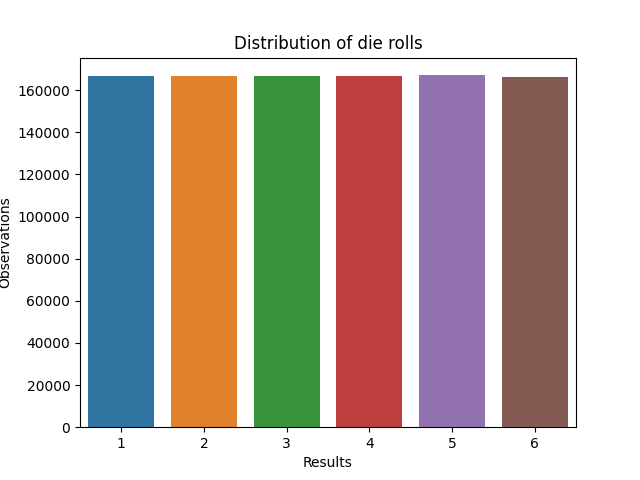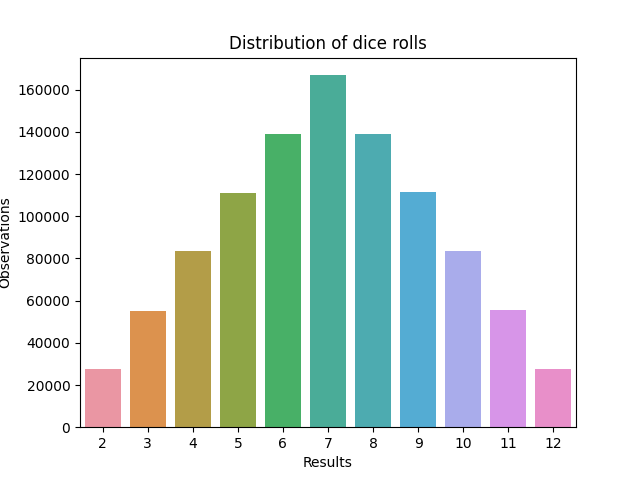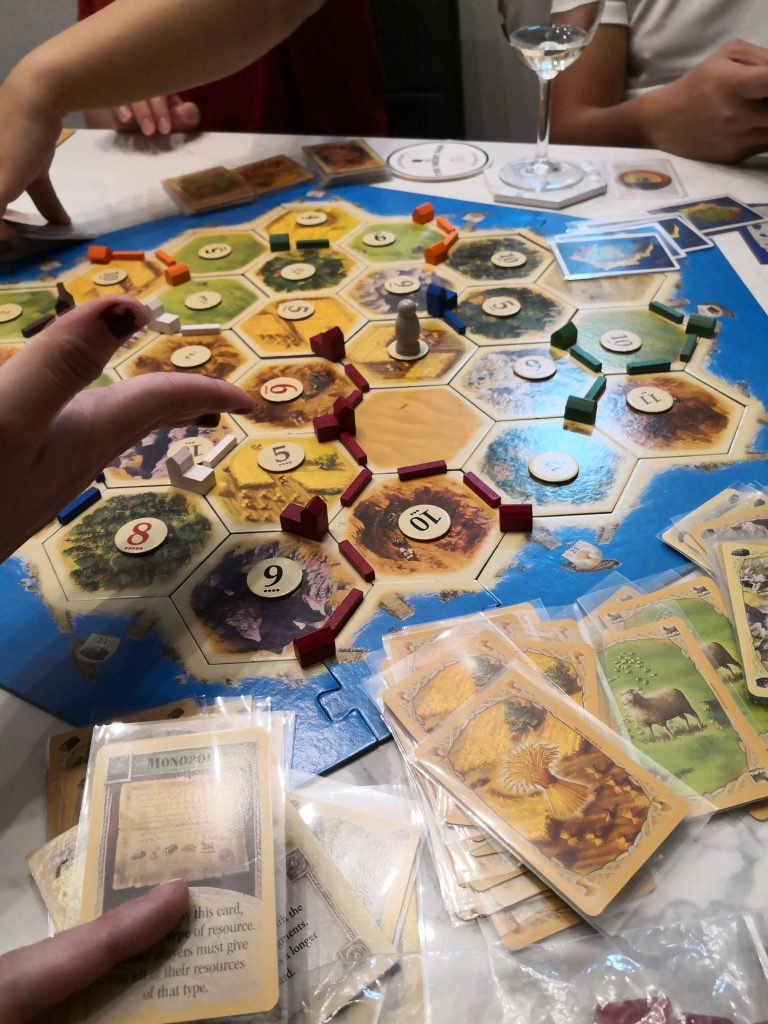# Rolling Pythonic dice for Catan

I got introduced to Catan several weeks ago. I think it is the best board game I’ve ever played. Its mechanics and gameplay appealed to me with a nice blend of strategy and player-involvement. Even if one player falls behind, they can still influence the gameplay.

However, there is one aspect of such games that I didn’t like: Rolling dice.

We’ve all experienced a wayward die (or two) falling and knocking over set pieces. I suggested to drop the dice and roll them in a bowl. I had seen this practice during Chinese New Year when my relatives played games with dice. They would drop the dice into a big white porcelain bowl. One die would hit the others, induce more randomness, and thereby influence the outcome–or so I thought.

Anyway, back to Catan, we ended up dropping our dice in front of us and trying not to knock over our settlements while occasionally picking up fallen die on the floor.

So thinking of that problem, I wrote a simple Python script to roll 2 dice.

``````import random

dice1, dice2 = random.randint(1, 6), random.randint(1, 6)
dice_sum = dice1 + dice2

def dice_print():
print(f"{dice1} and {dice2} were rolled")
print(f"{dice_sum} is the rolled total\n")

dice_print()``````

Running the program outputs the following:

``````5 and 3 were rolled
8 is the rolled total``````

Now, this isn’t a truly random number generator. The program most certainly is not even unique. But, it’ll do for people like me who want to roll virtual dice and not have Catanic earthquakes happen every round. Online dice rollers are useful; though, I suppose people want to feel like they own the outcome by rolling the dice themselves.

How would the probability distribution look like, I wondered. No matter, it was a simple case of writing more lines to roll the dice a million times. With Seaborn, these were the results of a single die and two dice.

``````dice_roll_count = 0
dice_roll_max = 1000000
dice1_rolled, dice2_rolled, dice_sum_rolled = [], [], []

while dice_roll_count  < dice_roll_max:
dice1 = random.randint(1, 6)
dice2 = random.randint(1, 6)
dice2_rolled.append(dice2)
dice1_rolled.append(dice1)
dice_sum = dice1 + dice2
dice_sum_rolled.append(dice_sum)
dice_roll_count += 1

data_dice1 = sns.countplot(dice1_rolled).set(title="Distribution of die rolls", xlabel="Results", ylabel="Observations")
plt.show()

data_dice_sum = sns.countplot(dice_sum_rolled).set(title="Distribution of dice rolls", xlabel="Results", ylabel="Observations")
plt.show()``````

Note to self to use list comprehensions to further shorten the code.One does seem to have a 1 in 6 chance of getting these outcomes.The sum of rolling 2 dice follows the dots on the Catan tokens following the probability of the outcomes.The GF (to my left) probably wanting to wreck my settlements after I played the Monopoly card to grab everyone’s wheat even after I had a natural wheat monopoly.

But no matter, a win is a win. Catan is a nice board game and I readily recommend it to anyone. Also, Python is a nice programming language. Anyone can pick it up.

Couple of days since I wrote the above program, I refined it. This lets me roll one die n times.

``````import random

die_start, die_end = 1, 6

def roll(die, print_out):

def roll_die(print_out):
result = random.randint(die_start, die_end)
if print_out == 1:
print(f"{result} was rolled")
return result
else:
return result

if die == 0:
return
else:
count_die, sum_of_rolls = 0, 0
while count_die < die:
sum_of_rolls += roll_die(print_out)
count_die += 1
print(f"((( {sum_of_rolls} ))) was rolled")

roll(2, 1)``````

Running `roll(2, 1)` outputs the results of two rolls and their sum.

``````1 was rolled
2 was rolled
((( 3 ))) was rolled``````

Running `roll(2, 0) `squelches the individual results and ouputs just the sum of rolled totals. I like it. 🙂

``((( 3 ))) was rolled``